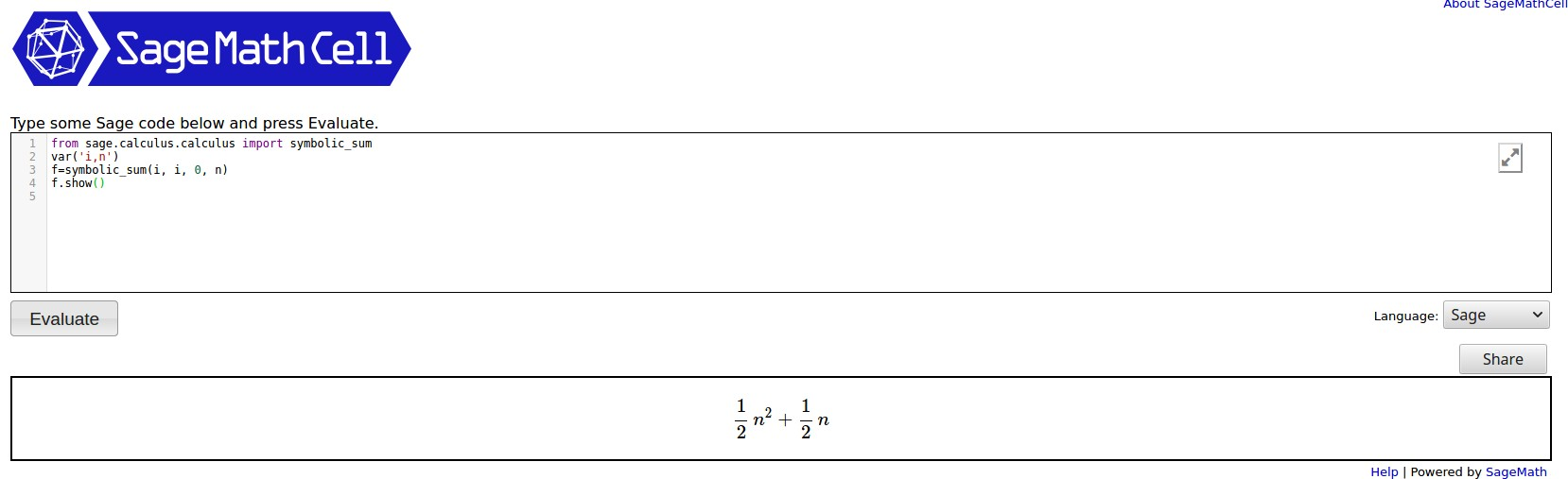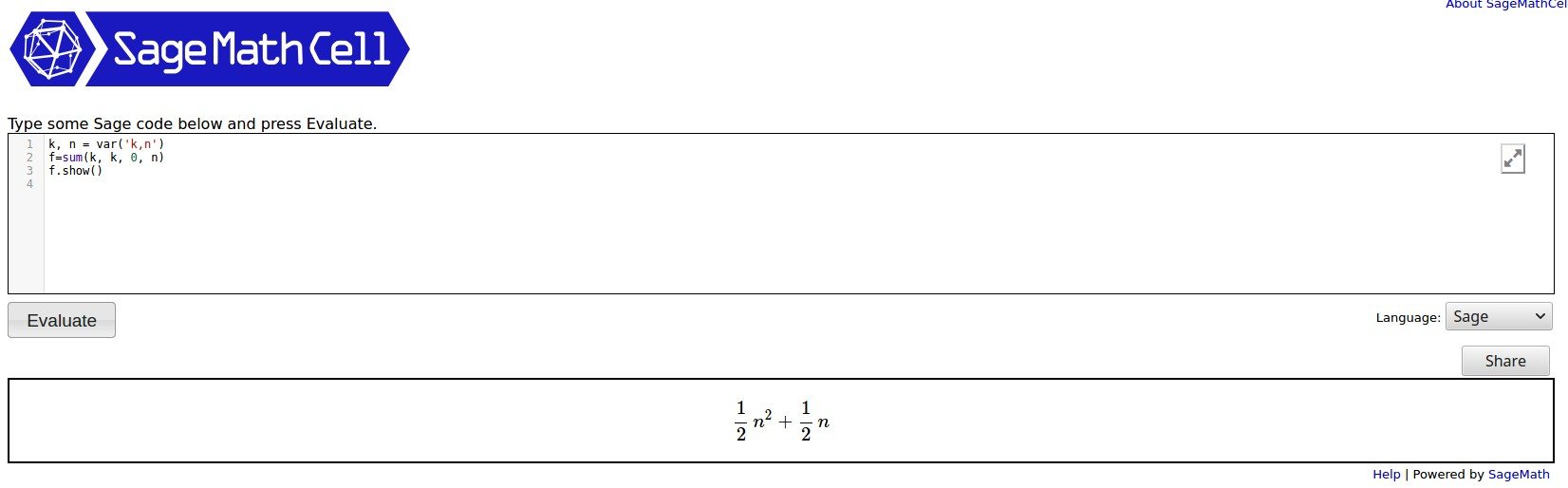# Revision history [back]

If you want a symbolic sum you should use symbolic_sum, which you can find documented here. Here is an example:

from sage.calculus.calculus import symbolic_sum
var('i,n')
f=symbolic_sum(i, i, 0, n)
f.show()


The output, running in a SageCellServer is shown below:If you want a symbolic sum you should have 2 choices. First, using sum:

i, n = var('i,n')
f=sum(i, i, 0, n)
f.show()


The output, running in a SageCellServer is shown below:You can find the documentation here.

If you use symbolic_sum, which you can find documented here. Here is an example:, then you could try:

from sage.calculus.calculus import symbolic_sum
var('i,n')
f=symbolic_sum(i, i, 0, n)
f.show()


The output, running in a SageCellServer is shown below: output is:If you want a symbolic sum you have 2 choices. choices that I know of. First, using sum:

i, n = var('i,n')
f=sum(i, i, 0, n)
f.show()


The output, running in a SageCellServer is shown below:You can find the documentation here.

If you use symbolic_sum, documented here, then you could try:

from sage.calculus.calculus import symbolic_sum
var('i,n')
f=symbolic_sum(i, i, 0, n)
f.show()


The output is:If you want a symbolic sum you have 2 choices that I know of. First, using sum:sum:

i, n = var('i,n')
f=sum(i, i, 0, n)
f.show()


The output, running in a SageCellServer is shown below:You can find the documentation here.

If you use symbolic_sum, documented here, then you could try:

from sage.calculus.calculus import symbolic_sum
var('i,n')
f=symbolic_sum(i, i, 0, n)
f.show()


The output is:If you want a symbolic sum you have 2 choices that I know of. First, using sum:

i, k, n = var('i,n')
f=sum(i, i, f=sum(k, k, 0, n)
f.show()


The output, running in a SageCellServer is shown below:You can find the documentation here.

If you use symbolic_sum, documented here, then you could try:

from sage.calculus.calculus import symbolic_sum
var('i,n')
f=symbolic_sum(i, i, 0, n)
f.show()


The output is:If you want a symbolic sum you have 2 choices that I know of. First, using sum:

k, n = var('i,n')
var('k,n')
f=sum(k, k, 0, n)
f.show()


The output, running in a SageCellServer is shown below:You can find the documentation here.

If you use symbolic_sum, documented here, then you could try:

from sage.calculus.calculus import symbolic_sum
var('i,n')
f=symbolic_sum(i, i, 0, n)
f.show()


The output is: